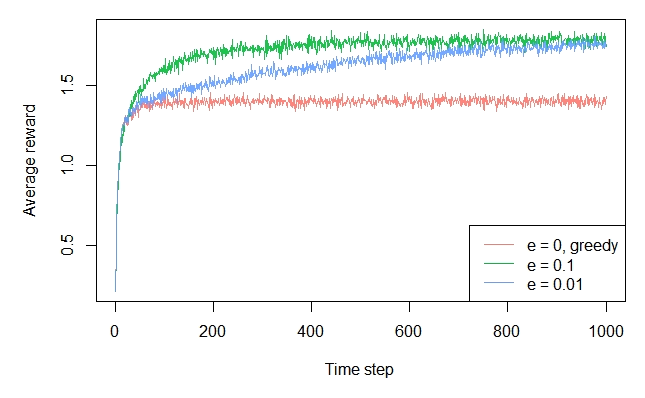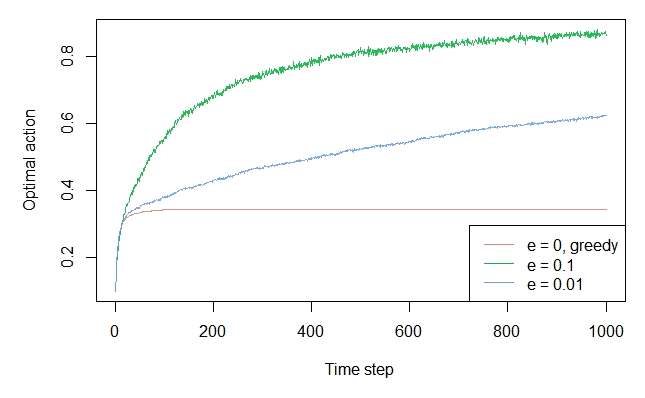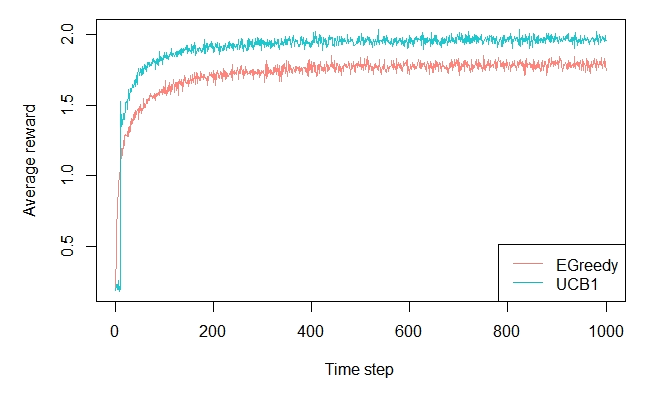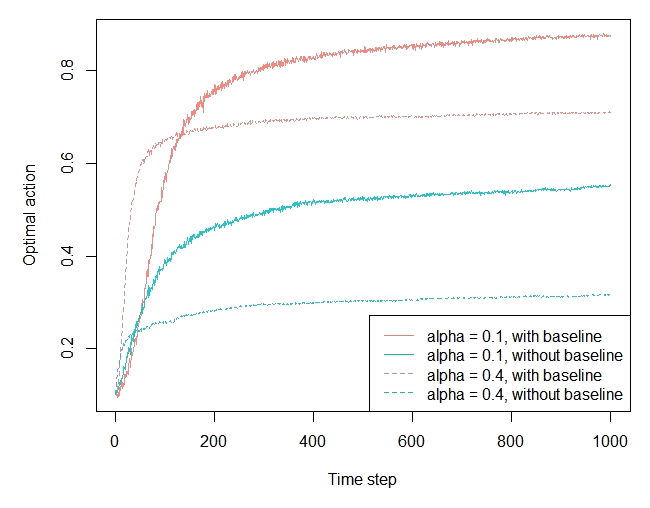# Demo: Replication Sutton & Barto, Reinforcement Learning: An Introduction, Chapter 2

#### 2019-03-17

Simulation of the multi-armed Bandit examples in chapter 2 of “Reinforcement Learning: An Introduction” by Sutton and Barto, 2nd ed. (Version: 2018)

This book is available here: Sutton&Barto

# 2.3 The 10-armed Testbed

Generate the 10 arms.


library(contextual)

set.seed(2)
mus             <- rnorm(10, 0, 1)
sigmas          <- rep(1, 10)
history         <- simulator$run() plot(history, type = "average", regret = FALSE, lwd = 1, legend_position = "bottomright") plot(history, type = "optimal", lwd = 1, legend_position = "bottomright")# 2.6 - Optimistic values  agents <- list(Agent$new(EpsilonGreedyPolicy$new(0), bandit, "optimistic greedy Q0 = 5, e = 0"), Agent$new(EpsilonGreedyPolicy$new(0.1), bandit, "realistic greedy Q0 = 0, e = 0.1")) agents[]$policy$theta$mean <- as.list(rep(5,10))
agents[]$policy$theta$n <- as.list(rep(5,10)) simulator <- Simulator$new(agents = agents, horizon = 1000, simulations = 2000)
history         <- simulator$run() plot(history, type = "average", regret = FALSE, lwd = 1, legend_position = "bottomright")# 2.8 - Gradient Bandit Algorithms set.seed(2) mus <- rnorm(10, 0, 1) sigmas <- rep(1, 10) bandit <- BasicGaussianBandit$new(mu_per_arm = mus, sigma_per_arm = sigmas,
mu_offset = 4)

agents          <- list(Agent$new(GradientPolicy$new(0.1, TRUE),  bandit,
"alpha = 0.1, with baseline"),
Agent$new(GradientPolicy$new(0.1, FALSE), bandit,
"alpha = 0.1, without baseline"),
Agent$new(GradientPolicy$new(0.4, TRUE),  bandit,
"alpha = 0.4, with baseline"),
Agent$new(GradientPolicy$new(0.4, FALSE), bandit,
"alpha = 0.4, without baseline"))

simulator       <- Simulator$new(agents = agents, horizon = 1000, simulations = 2000) history <- simulator$run()

plot(history, type = "optimal", lwd = 1, legend_position = "bottomright",
color_step = 2, lty_step = 2)